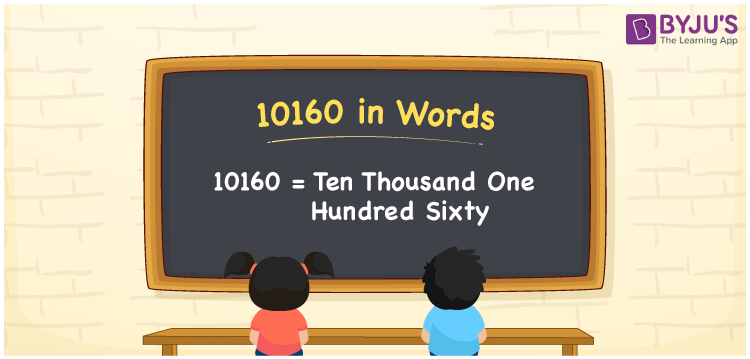# 10160 in words

10160 in words is written as Ten Thousand One Hundred and Sixty. In 10160, first 1 has a place value of ten thousand, second 1 has a place value of hundred and 6 is in the place value of ten. The article on Place Value gives more information. The number 10160 is used in expressions that relate to money, distance, length, social media views, and many more. For example, “Yesterday’s step count during my evening walk was Ten Thousand One Hundred and Sixty?.”

 10160 in words Ten Thousand One Hundred and Sixty Ten Thousand One Hundred and Sixty in Numbers 10160

## 10160 in English Words## How to Write 10160 in Words?

We can convert 10160 to words using a place value chart. This can be done as follows. The number 10160 has 5 digits, so let’s make a chart that shows the place value up to 5 digits.

 Ten thousand Thousands Hundreds Tens Ones 1 0 1 6 0

Thus, we can write the expanded form as:

1 × Ten thousand + 0 × Thousand + 1 × Hundred + 6 × Ten + 0 × One

= 1 × 10000 + 0 × 1000 + 1 × 100 + 6 × 10 + 0 × 1

= 10160.

= Ten Thousand One Hundred and Sixty.

10160 is the natural number that is succeeded by 10159 and preceded by 10161.

10160 in words – Ten Thousand One Hundred and Sixty.

Is 10160 an odd number? – No.

Is 10160 an even number? – Yes.

Is 10160 a perfect square number? – No.

Is 10160 a perfect cube number? – No.

Is 10160 a prime number? – No.

Is 10160 a composite number? – Yes.

## Solved Example

1. Write the number 10160 in expanded form

Solution: 1 x 10000 + 0 x 1000 + 1 x 100 + 6 x 10 + 0 x 1

Or Just 1 x 10000 + 1 x 100 + 6 x 10

We can write 10160 = 10000 + 0000 + 100 + 60 + 0

= 1 x 10000 + 0 x 1000 + 1 x 100 + 6 x 10 + 0 x 1.

## Frequently Asked Questions on 10160 in words

Q1

### How to write the number 10160 in words?

10160 in words is written as Ten Thousand One Hundred and Sixty.
Q2

### Is 10160 divisible by 3?

No. 10160 is not divisible by 3.
Q3

### Is 10160 a prime number?

No. 10160 is not a prime number.